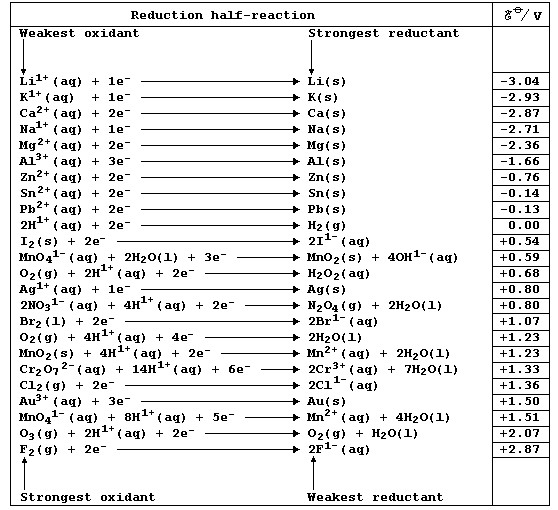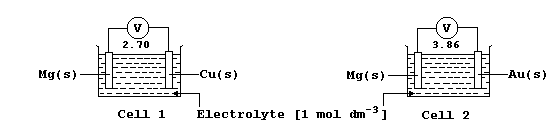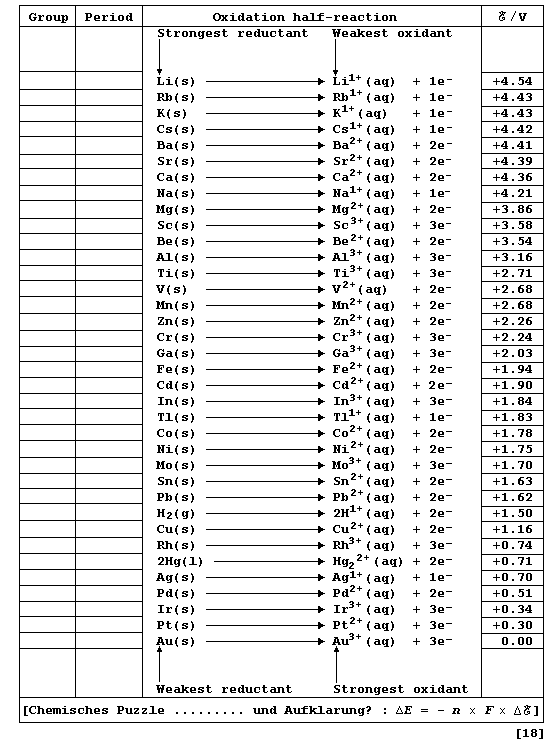```REFERENCE SECTION: REDOX POTENTIALS
Chemistry texts are often peppered with statements of the following
type: 'potassium is a strong reducing agent'; 'calcium is a stronger
reducing agent than magnesium'; 'acidified potassium manganate is a
strong oxidizing agent'; and, 'fluorine is a stronger oxidizing agent
than chlorine'. The quantitative basis for these and other related
descriptions is the (continually expanding) set of physical quantities
known as reduction potentials; a critical compilation of these data
is: A. J. Bard et al., Standard Potentials in Aqueous Solution, Marcel
Dekker, New York, 1985. Numerical values of redox potentials are rarely
used in introductory courses, partly because their correct use is quite
complicated: nevertheless, a selected sub-set of values - as shown in
this first Table - does provide a useful point of reference. [Reductant
and reducing agent are synonymous, as are oxidant and oxidizing agent.]``````#  These data have been obtained, under standardized conditions, using
sophisticated electrochemical cells. The diagram shows the basic design
of such cells; Cell 1 would be suitable for impoverished mortals, but
the well-endowed (or alchemists) would surely prefer Cell 2.``````Chemically, a metallic element (M) is characterized by its tendency to
lose one or more electrons and form a positive ion; i.e., it is readily
oxidized (Olé!): M ——————® Mn+ + ne-.  One of several different methods
of quantitatively comparing metallic character involves using oxidation
potentials. In principle, these values can be determined by measuring
potential differences of simple electrochemical cells. However, because
published sources of reduction potentials contain data obtained under
standardized conditions, it is both convenient and self-consistent to
derive oxidation potentials from such sources. This derivation involved
changing the sign of each value, assigning an arbitrary value of 0.00 V
to a gold reference electrode, and then executing simple arithmetic.
Complete the Table below, which refers to data obtained under standard
conditions (pH = 0; 298 K), by inserting the group number and period of
each element and by indicating each transition metal with an asterisk.``````Dr. R. Peters                                            Contents' List
Selected Principles - Introduction (1)```